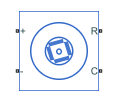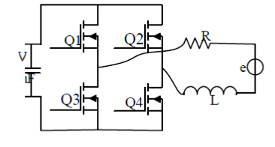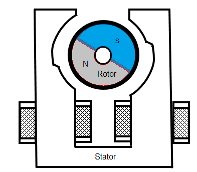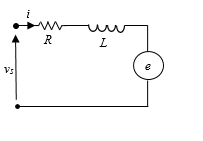# PMSM (Single-Phase)

Single-phase permanent magnet synchronous motor

•Libraries:
Simscape / Electrical / Electromechanical / Permanent Magnet

## Description

The PMSM (Single-Phase) represents a single-phase permanent magnet synchronous motor (PMSM), a type of DC motor that is useful for automation applications.

The figure shows the topology of the single-phase PMSM drive.The figure shows the motor construction with a single pole-pair on the rotor. Single-phase PMSMs are not self-starting unless the air gap is asymmetrical.The figure shows the equivalent circuit for the PMSM (Single-Phase) block.### Equations

The motor voltage equations are

`${v}_{s}=Ri+L\frac{di}{dt}+e$`

and

`${v}_{s}={V}_{m}\text{sin}\left({\omega }_{s}t+\epsilon \right),$`

where:

• vs is the supply voltage.

• i is the instantaneous motor current.

• R is the resistance of the windings.

• L is the self-inductance of the windings.

• e is the back-electromotive force (BEMF).

• ɷs is the angular frequency of the supply voltage.

• ε is the angle of the supply voltage.

The back electro-motive force (BEMF) is

`$e={k}_{e}{\omega }_{e}\text{sin}\left({\theta }_{e}\right),$`

where:

• ɷe is the rotor electrical angular velocity.

• θe is the rotor electrical angle.

• ke is the BEMF constant.

Due to the large low-permeability gaps between the stator and rotor, the saturation can be neglected. Therefore, the electric torque equations are

`${T}_{e}=p\text{\hspace{0.17em}}i\text{\hspace{0.17em}}{\psi }_{m}\text{sin}\left({\theta }_{e}\right)$`

and

`${\psi }_{m}=\frac{{k}_{e}}{p},$`

where:

• Te is the electric torque.

• ψm is the permanent magnet flux linkage.

• p is the number of pole pairs.

The mechanical equation is

`${J}_{m}\frac{d{\omega }_{r}}{dt}={T}_{e}-{T}_{L}-{B}_{m}{\omega }_{r},$`

where:

• Jm is the rotor inertia.

• TL is the torque load.

• Bm is the friction coefficient.

• ɷr is the rotor mechanical angular velocity.

### Model Thermal Effects

You can expose thermal ports to simulate the effects of generated heat and motor temperature. To expose the thermal ports and the Thermal parameters, set the Modeling option parameter to either:

• `No thermal port` — The block does not contain thermal ports.

• `Show thermal port` — The block contains thermal conserving ports for the stator and the rotor.

For more information about using thermal ports in actuator blocks, see Simulating Thermal Effects in Rotational and Translational Actuators.

### Variables

To set the priority and initial target values for the block variables before simulation, use the Initial Targets section in the block dialog box or Property Inspector. For more information, see Set Priority and Initial Target for Block Variables.

Nominal values provide a way to specify the expected magnitude of a variable in a model. Using system scaling based on nominal values increases the simulation robustness. You can specify nominal values using different sources, including the Nominal Values section in the block dialog box or Property Inspector. For more information, see System Scaling by Nominal Values.

## Limitations and Assumptions

• The machine air gap is free of saliency effects.

• The stator current has negligible effect on the flux distribution under normal operating conditions.

• The hysteresis, saturation effects, and eddy currents are neglected.

## Ports

### Conserving

expand all

Mechanical rotational conserving port associated with the machine rotor.

Mechanical rotational conserving port associated with the machine case.

Electrical conserving port associated with the supply positive terminal.

Electrical conserving port associated with the supply negative terminal.

Thermal conserving port associated with the stator.

#### Dependencies

To enable this port, set Modeling option to ```Show thermal port```.

Thermal conserving port associated with the rotor.

#### Dependencies

To enable this port, set Modeling option to `Show thermal port`.

## Parameters

expand all

Whether to enable the thermal ports of the block and model the effects of generated heat and motor temperature.

### Main

Number of permanent magnet pole pairs on the rotor.

Method for parameterizing the stator.

#### Dependencies

Selecting `Specify flux linkage` exposes the Permanent magnet flux linkage parameter.

Selecting `Specify back EMF constant` exposes the Back EMF constant parameter.

#### Dependencies

Selecting `Specify flux linkage` for the Permanent magnet flux linkage parameterization parameter exposes the Permanent magnet flux linkage parameter.

Back-electromotive force constant.

#### Dependencies

Selecting `Specify back EMF constant` for the Permanent magnet flux linkage parameterization parameter exposes the Back EMF constant parameter.

The direct-axis inductance.

Resistance of each of the stator windings.

Rotor angular position at standstill due to asymmetric air gap.

### Mechanical

Inertia of the rotor attached to mechanical translational port R.

Damping of the rotor.

### Thermal

To enable these parameters, set Modeling option to `Show thermal port`.

Temperature for which motor parameters are quoted.

Coefficient α in the equation relating resistance to temperature for all three windings, as described in Thermal Model for Actuator Blocks. The default value, `3.93e-3` 1/K, is for copper.

Fractional rate of change of permanent magnet flux density with temperature. Use this parameter to linearly reduce the torque and the induced back EMF as temperature rises.

Thermal mass value for each stator winding. The thermal mass is the energy required to raise the temperature by one degree.

Thermal mass of the rotor. The thermal mass is the energy required to raise the temperature of the rotor by one degree.

 Ertugrul, N. and C. Doudle. “Dynamic analysis of a single-phase line-starting permanent magnet synchronous motor.” Proceedings of International Conference on Power Electronics, Drives and Energy Systems for Industrial Growth. Vol. 1, 1996, pp. 603–609.